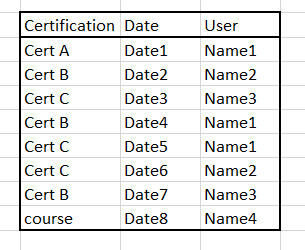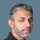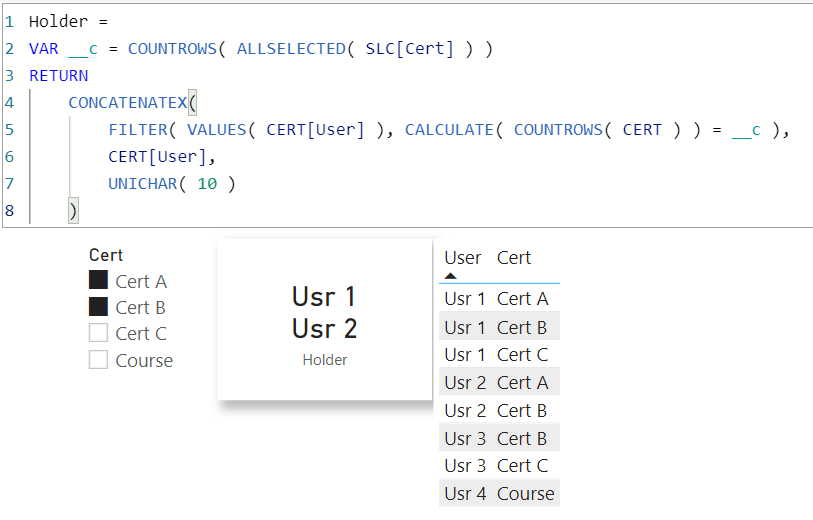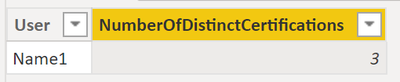cancel
Showing results for
Did you mean:Super User

## Filtering

Hello Experts,

Through the following table, I am looking for 3 tables by DAX.

1- A table that shows all users that have 3 certifications A,B,C (complete certification)

2 A table that shows the users and their certifications and missed certifications

3- A table that shows users that have not any certification.2 ACCEPTED SOLUTIONSSuper User

@koorosh

Here are table codes

``````EVALUATE
FILTER (
VALUES ( Table1[User] ),
COUNTROWS (
INTERSECT (
CALCULATETABLE ( VALUES ( Table1[Certification] ) ),
{ "Cert A", "Cert B", "Cert C" }
)
) = 3
)

EVALUATE
CROSSJOIN (
VALUES ( Table1[user] ),
SELECTCOLUMNS ( { "Cert A", "Cert B", "Cert C" }, "Cert", [Value] )
),
"Status",
VAR _cert = [Cert]
RETURN
IF (
CALCULATE ( ISEMPTY ( FILTER ( table1, table1[Certification] = _cert ) ) ),
"Missing",
"Certified"
)
)

EVALUATE
FILTER (
VALUES ( Table1[User] ),
COUNTROWS (
INTERSECT (
CALCULATETABLE ( VALUES ( Table1[Certification] ) ),
{ "Cert A", "Cert B", "Cert C" }
)
) = 0
)``````

Check the attached file below my signature

Did I answer your question? Mark my post as a solution! and hit thumbs upSuper UserThanks to the great efforts by MS engineers to simplify syntax of DAX! Most beginners are SUCCESSFULLY MISLED to think that they could easily master DAX; but it turns out that the intricacy of the most frequently used RANKX() is still way beyond their comprehension! DAX is simple, but NOT EASY!
6 REPLIES 6Super UserThanks to the great efforts by MS engineers to simplify syntax of DAX! Most beginners are SUCCESSFULLY MISLED to think that they could easily master DAX; but it turns out that the intricacy of the most frequently used RANKX() is still way beyond their comprehension! DAX is simple, but NOT EASY!Super User

Hi @koorosh ,

Here the third one:```Table3 =
FILTER (
SUMMARIZE (
'Table',
Table[User],
"NumberOfDistinctCertifications",
CALCULATE (
DISTINCTCOUNT ( Table[Certification] ), Table[Certification] in {"Cert A", "Cert B", "Cert C"} ),
"NumberOfDistinctCourses",
CALCULATE (
DISTINCTCOUNT ( Table[Certification] ), Table[Certification] in {"course"} )
),
[NumberOfDistinctCourses] > 0 && [NumberOfDistinctCertifications] < 1
)```

Does this help? 🙂

Proud to be a Super User!Super User

@koorosh

Here are table codes

``````EVALUATE
FILTER (
VALUES ( Table1[User] ),
COUNTROWS (
INTERSECT (
CALCULATETABLE ( VALUES ( Table1[Certification] ) ),
{ "Cert A", "Cert B", "Cert C" }
)
) = 3
)

EVALUATE
CROSSJOIN (
VALUES ( Table1[user] ),
SELECTCOLUMNS ( { "Cert A", "Cert B", "Cert C" }, "Cert", [Value] )
),
"Status",
VAR _cert = [Cert]
RETURN
IF (
CALCULATE ( ISEMPTY ( FILTER ( table1, table1[Certification] = _cert ) ) ),
"Missing",
"Certified"
)
)

EVALUATE
FILTER (
VALUES ( Table1[User] ),
COUNTROWS (
INTERSECT (
CALCULATETABLE ( VALUES ( Table1[Certification] ) ),
{ "Cert A", "Cert B", "Cert C" }
)
) = 0
)``````

Check the attached file below my signature

Did I answer your question? Mark my post as a solution! and hit thumbs upSuper User

Thanks Mohammed, If the excel data source has more columns and I want to include them in the T1 and T2, wherein Dax for T1 and T2, I should bring them? For example name of the company of each user and the date of each certification.Super User

@koorosh

Yes, you can do it by using these tables as filters in a CALCULATEDTABLE function.

Did I answer your question? Mark my post as a solution! and hit thumbs upSuper User

Hi @koorosh ,

Here the first one:```Table1 =FILTER (
SUMMARIZE (
FILTER  ( 'Table', Table20[Certification] in {"Cert A", "Cert B", "Cert C"} ),
Table[User],
"NumberOfDistinctCertifications", DISTINCTCOUNT ( Table[Certification] )
),
[NumberOfDistinctCertifications] = 3
)```

Does this help? 🙂

Proud to be a Super User!